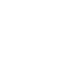# Feb 27 ACT & SAT Question of the Day

http://sat.collegeboard.org/practice/sat-question-of-the-day?src=R&questionId=20130227 (This link takes you to today’s question. If you use my archive, you will see the question related to my SAT explanation for that date.)

The answer is A.  This is a tough question for most students and the evidence is in the fact that as of right now only 36% are getting it right.  I don’t think the SAT staff’s explanation is much help   It is one of those that my students will say that the explanation is harder than the question.  I did this question in about 15 seconds with one of my favorite “Wizard Strategies” from page 21 in my manual “Demystifying the SAT and ACT.”  My students know I like to call it, “Change the abstract to the concrete.”  That is, when the test writer, your opponent, doesn’t give you numbers but gives you rules about the variables, you just get to make stuff up!!

They tell you that a/b is 3.  So, that’s a=3 and b=1 or 3/1.  Then they give you b/c =7.  Since you already made b=1, then c has to be 1/7 because b has to be 7 times as big as c.  Using our real numbers a+b is 3+1 or 4 in the numerator.  b+c is 1 + 1/7 or 8/7 (1.14) in the denominator.  Now divide and you get 3.50, answer A.  Shazaam!

Now, I’ve got to tell you, I’m lazy and don’t like to use fractions and I saw a shortcut that made everything integers so I could do all this in my head.  Did you see it?  I eliminated having to deal with 1/7 by multiplying my original numbers in the example above by 7.  That gave me a/b=21/7 and b/c=7/1.  Then when I added, a+b=21+7=28.  b+c=7+1=8.  So, 28/8=3.5 and I’m done.  Another “shazaam” with a bonus.

Hope that helps.

Whose explanation do you like?  Their explanation or mine?  Send me an email and let me know.  I won’t be offended.  I’m just interested in knowing how many math whizzes we have out there and how many test-taking strategists are reading my blog.

Let’s see what the ACT folks have in store for us this mornig.

http://www.act.org/qotd/ (The ACT staff does not put a date on their questions so if you click on an archived blog, you’ll get today’s question and the old explanation. Sorry. The SAT staff has dated their questions; so, the archive is helpful. The ACT folks simply don’t do that.)-

The answer is B.  After doing that SAT question, this is a mercifully easy question.  Most of you can do this one since you learned to raise x to a power in pre-algebra.  Multiplying x times itself four times is x to the fourth power, x4.

But let’s say you didn’t remember that.  So, what could you do?  Just do what we did for the last question: change the abstract to the concrete.  Let’s use 2 for x.  2 times 2 times 2 times 2 is 16.  Then plug 2 into x for all the answers.  For example, Answer A becomes 4 times 2 which is 8 and that’s wrong because we know the answer has to be 16 based on our substitution of  2 for x.  B has to be right since we know that 2 to the fourth power is 16.  The other answers are wrong when we plug in 2 for x.  Look at C, 2+4 is 6 and so on.

There are a lot of these kinds of questions on the test ranging from easy (like this ACT question) to hard (like this SAT question).  No matter.  You can always “substitute the abstract for the concrete” when they don’t give you numbers and only give you variables.  Just make stuff up by following their rules.

This was a magical morning.  It’s almost like the SAT and the ACT folks were collaborating (working together) with me since they each gave me a question to talk about this strategy — an easy one and a hard one.  Nope, we aren’t in cahoots (working together).  The stars were just lined up and this fell into place.

Hey, here’s a big shout out to my new students at WOHS!!  Welcome aboard.

The Wizard